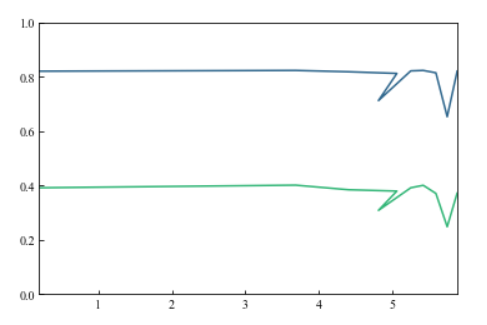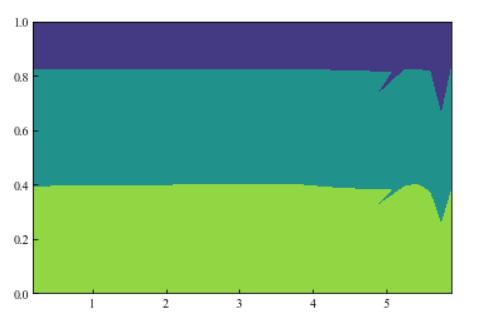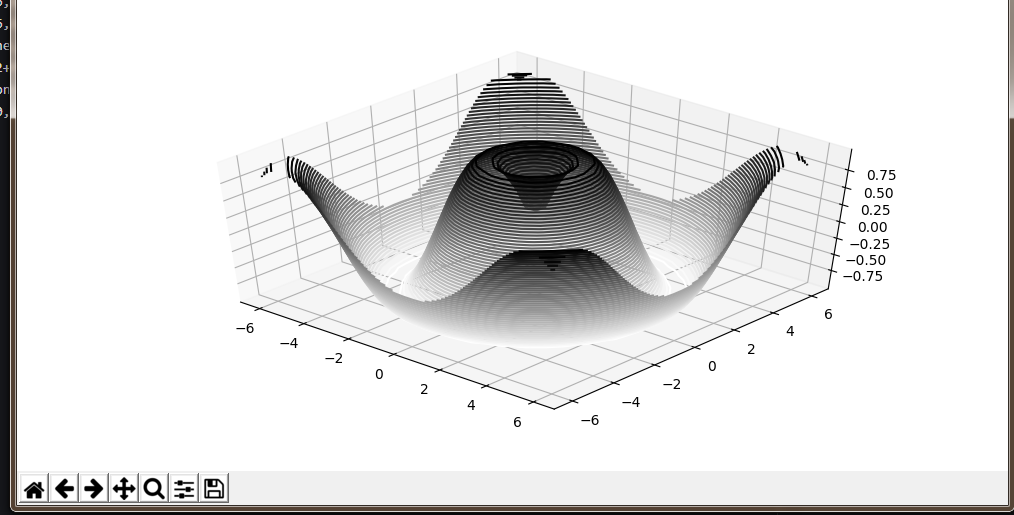• 下面是绘制的效果 这里是绘制的代码 # -*- coding: utf-8 -*- import numpy as np import pandas as pd import matplotlib.pyplot as plt # 计算x,y坐标对应的高度值 def f(x,y): #the height function return x ...

下面是绘制的效果这里是绘制的代码

# -*- coding: utf-8 -*-
import numpy as np
import pandas as pd
import matplotlib.pyplot as plt

# 计算x,y坐标对应的高度值
def f(x,y):
#the height function
return x * x + y * y

# 生成x,y的数据
x = np.linspace(-3, 3, 7)
y = np.linspace(-3, 3, 7)
print("x=",x)
print("y=",y)

# 把x,y数据生成mesh网格状的数据，因为等高线的显示是在网格的基础上添加上高度值
X, Y = np.meshgrid(x, y)
print("X=",X)
print("Y=",Y)

# 填充等高线
c = plt.contour(X, Y, f(X, Y))
print("Z=",f(X, Y))

# 等高线标签
plt.clabel(c,inline=True,fontsize=10)
# 显示图表
plt.show()

输出控制台的数据

x= [-3. -2. -1.  0.  1.  2.  3.]
y= [-3. -2. -1.  0.  1.  2.  3.]
X= [[-3. -2. -1.  0.  1.  2.  3.]
[-3. -2. -1.  0.  1.  2.  3.]
[-3. -2. -1.  0.  1.  2.  3.]
[-3. -2. -1.  0.  1.  2.  3.]
[-3. -2. -1.  0.  1.  2.  3.]
[-3. -2. -1.  0.  1.  2.  3.]
[-3. -2. -1.  0.  1.  2.  3.]]
Y= [[-3. -3. -3. -3. -3. -3. -3.]
[-2. -2. -2. -2. -2. -2. -2.]
[-1. -1. -1. -1. -1. -1. -1.]
[ 0.  0.  0.  0.  0.  0.  0.]
[ 1.  1.  1.  1.  1.  1.  1.]
[ 2.  2.  2.  2.  2.  2.  2.]
[ 3.  3.  3.  3.  3.  3.  3.]]
Z= [[18. 13. 10.  9. 10. 13. 18.]
[13.  8.  5.  4.  5.  8. 13.]
[10.  5.  2.  1.  2.  5. 10.]
[ 9.  4.  1.  0.  1.  4.  9.]
[10.  5.  2.  1.  2.  5. 10.]
[13.  8.  5.  4.  5.  8. 13.]
[18. 13. 10.  9. 10. 13. 18.]]

通过数据可以看出，越靠边缘的数据越大，最小的数位于中间

展开全文• python绘制等高线

千次阅读 2019-06-19 17:49:28
https://blog.csdn.net/Mr_Cat123/article/details/80677525 python等高线色阶 https://matplotlib.org/users/colormaps.html
展开全文• plt.contour(X,Y,Z,levels=2) X，Y ： 坐标 X和Y必须都是2-D，且形状与Z相同 或者它们必须都是1-d，这样len(X)=M...levels： int或类似数组，确定轮廓线/区域的数量和位置。 plt.contourf(X,Y,Z,levels=2) ...
plt.contour(X,Y,Z,levels=2)

• X，Y ： 坐标
• X和Y必须都是2-D，且形状与Z相同
• 或者它们必须都是1-d，这样len(X)=M是Z中的列数len(Y)=N是Z中的行数
• Z ： array-like（N，M），绘制轮廓的高度值
• levels： int或类似数组，确定轮廓线/区域的数量和位置。plt.contourf(X,Y,Z,levels=2)展开全文python
• python绘制等高线图

千次阅读 2019-02-25 22:25:58
# encoding: utf-8 import numpy as np import pandas as pd import matplotlib.pyplot as plt from mpl_toolkits import mplot3d fig=plt.figure() x_line=np.linspace(-6,6,30) y_line=np.linspace(-6,6,30) ...
# encoding: utf-8
import numpy as np
import pandas as pd
import matplotlib.pyplot as plt
from mpl_toolkits import mplot3d
fig=plt.figure()
x_line=np.linspace(-6,6,30)
y_line=np.linspace(-6,6,30)
X,Y=np.meshgrid(x_line,y_line)
Z=np.sin(np.sqrt(X**2+Y**2))
ax=plt.axes(projection='3d')
ax.contour3D(X,Y,Z,50,cmap='binary')
plt.show()展开全文• Python matplotlib 绘制等高线图

千次阅读 2021-11-20 14:44:28
前言 我们在往期对matplotlib.pyplot()方法学习，到现在我们已经会... 这么详细的Python matplotlib 绘制图形 还不赶紧收藏_ 超详细的Python matplotlib 绘制直方图 赶紧收藏_ 超详细的Python matplotlibpython 开发语言 后端
• Python画等高线

千次阅读 2017-11-27 14:41:22
# 绘制等高线数据 plt.clabel(C, inline = True, fontsize = 10) # 去除坐标轴 plt.axis('off') plt.tick_params(axis='both', left='off', top='off', right='off', bottom='off', labelleft='off', labeltop='off...
• 如何根据离散点自动绘制等值线(等高线)之客观分析另一种离散点分析等值线的方法是将离散的资料分析到网格点上然后进行格点的曲线拟合或者等值线追踪。常用的插值方案很多，一般来说比较精确的是逐步订正法和最优插值...
• python matplotlib contour画等高线图

万次阅读 多人点赞 2019-08-26 14:06:07
由于这里是有四个变量，x,y,z,和f,而x+y+z=163,需要做的是画出横坐标为x,纵坐标为y，高为f的等高线图，跟上面的例子已经不同，上面的例子只有三个变量，x,y,和z，画出x为横坐标，y为纵坐标，z为高的图，所以两者是...
• Python画等高线以及标注

千次阅读 2021-11-16 09:47:08
等高线（Contour Line）是一种描述地貌的主要形式，是指在地图上高程相等的相邻各点所连成的闭合曲线。将海拔高度相同的点，垂直投影到一个水平面，并按比例缩绘在图纸上，就得到了等高线。 import numpy as np ...Python等高线
• python matplotlib 绘制等高线 """ 绘制等高线 """ import numpy as np import matplotlib.pyplot as mp # 准备数据 num = 500 x, y = np.meshgrid(np.linspace(-3, 3, num), np.linspace(-3, 3, num)) print(x...
• 已知三维坐标和高度值的关系后，如何绘制出三维等高线图。 注：高度值由三维坐标计算得出python
• 我试图产生一个等高线图所说的标量，使它看起来有点像附加的图片使用ParaView。我宁愿使用vtk库而不是使用Matplotlib之类的东西，因为我认为它们可以产生更好的可视化效果。在我在网上看了几个例子，但没有一个对我...
• python等高线

2020-11-23 18:47:26
我知道如何从文本文件中提取数据，但我不知道如何在基于数据列的等高线图中绘制图形。 因此，列由; 分隔。第一列是半径，第二列是高度，第三列是k_eff。 74.5;160.0;2.1744583.0;177.0;2.188395.5;202.0;2. ...
• 用python的matplotlib画等高线

千次阅读 2018-08-11 14:47:32
python的matplotlib画等高线 对离散数据画等高线，填充颜色，并显示颜色条 生成网格，然后通过插值填充数据 对插值后的数据画等高线 根据最大值和最小值画colorbar 画等高线 # 产生网格，temp_data是n*3维...python matplotlib contourf 等高线 colorbar
• 小黄人番外短片合集 — 第14集【2015.12.08 Cro Minion 疯狂小黄人】 Matplotlib 系列文章（持续更新中）： ...《Python 数据分析三剑客之 Matplotlib（二）：文本描述 / 中文支持 / 画布 / 网格等基本图像...python 数据分析 matplotlib 等高线图 数据可视化
• np.vstack([m1, m2]) kernel = stats.gaussian_kde(values) Z = np.reshape(kernel(positions).T, X.shape) return X, Y, Z 然后，可以用^{}绘制X, Y, Z = density_estimation(m1, m2) fig, ax = plt.subplots() # ...
• python+matplotlib 绘制等高线步骤有七：有一个m*n维的矩阵(data)，其元素的值代表高度构造两个向量：x(1*n)和y(1*m)。这两个向量用来构造网格坐标矩阵(网格坐标矩阵m*n维，可见与data同)构造网格坐标矩阵X,Y进行...
• 密度和轮廓图有些情况下，我们需要在二维图表中使用轮廓或颜色区域来展示三维的数据(可以设想等高线地图或温度分布图)。Matplotlib 提供了三个有用的函数来处理这项任务：plt.contour绘制轮廓图plt.contourf来绘制...
• 用matplotlib画等高线图详解

千次阅读 2020-12-28 23:15:07
因为等高线的图有三个信息：x,y以及x,y所对应的高度值。这个高度值的计算我们用一个函数来表述：计算x,y坐标对应的高度值def f(x,y):return (1-x/2+x**5+y**3) * np.exp(-x**2-y**2)这个函数看起来挺复杂的，但我们...
• 主要介绍了Python绘制热力图,结合实例形式分析了Python使用pyheatmap及matplotlib模块进行数值计算与图形绘制相关操作技巧,需要的朋友可以参考下
• 我试图用Matplotlib在等高线绘制一些点。我有标量场，我想从中绘制轮廓。然而，myndarray的维数为0 x 20，但实际空间从-4到4不等。我可以用这段代码绘制这个等高线：x, y = numpy.mgrid[-4:4:20*1j, -4:4:20*1j]# ...
• 上回介绍 matplotlib 这个关键的库是画折线图的时候Mike：【python碎碎念】matplotlib 超好用的画图库​zhuanlan.zhihu.com今天我们还是用 matplotlib 这个库讲一讲等高线。...首先，当你绘制等高线的时候，你需要思...
• python matplotlib绘制等高线、等值线图 需求，是技术进步的原动力。先描述需求，并进行需求分析，再进行技术路线选型和编码实现。 一、需求描述 给附件1文件，是三列数据，想要如图这样的效果。 二、需求分析 这是...python 开发语言 后端
• Python之Matplotlib数据可视化(二)：密度图与等高线图用等高线图可视化三维数据用彩色等高线可视化三维数据带填充色的三维数据可视化图重新渲染三维数据的彩色图在彩色图上加上带数据标签的等高线备注有时在二维图上...
• 有了合适的数据之后，程序调用contour()函数绘制等高线，调用contourf()函数为等高线图填充颜色。在调用 contour()、contourf() 函数时可以指定如下常用参数：x：指定 X 轴数据。y：指定 Y 轴数据。z：指定 X、Y ......

python绘制等高线python 订阅